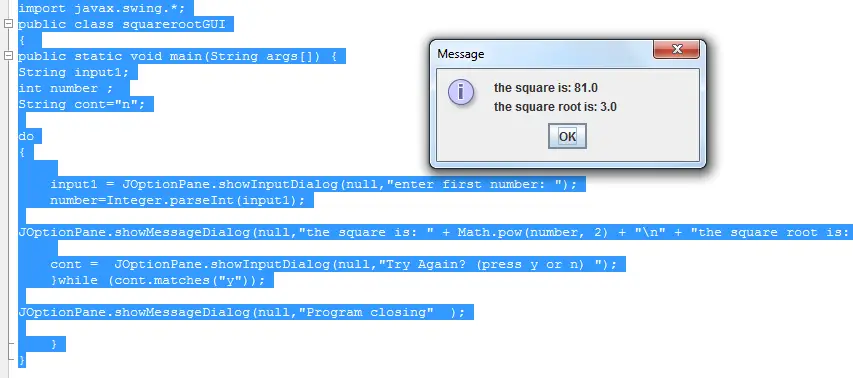# Get the Square and Square Root of a number in Java• Version
• File Size 3.14 KB
• File Count 1
• Create Date May 12, 2016
• Last Updated May 12, 2016

# Get the Square and Square Root of a number in Java

Get the Square and Square Root of a number in Java

This java program will accept an input from a user and then the program will display the square and the square root of the number entered by the user.

This is written in console and gui (graphical user interface) version.

Source code:

```import javax.swing.*;
public class squarerootGUI
{
public static void main(String args[]) {
String input1;
int number ;
String cont="n";

do
{

input1 = JOptionPane.showInputDialog(null,"enter first number: ");
number=Integer.parseInt(input1);

JOptionPane.showMessageDialog(null,"the square is: " + Math.pow(number, 2) + "
" + "the square root is: " + Math.sqrt(number) );

cont =	JOptionPane.showInputDialog(null,"Try Again? (press y or n) ");
}while (cont.matches("y"));

JOptionPane.showMessageDialog(null,"Program closing"  );

}
}
```